Next: Transverse-Field Measurements Up: SR Studies of the Previous: Experimental Setup   Contents

# Zero-Field Measurements

In this chapter, zero-field (ZF)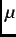SR measurements on the Pr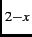Ce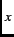CuO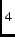single crystals are presented. For all measurements described here, the initial muon-spin polarization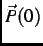was directed parallel to the CuO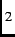layers.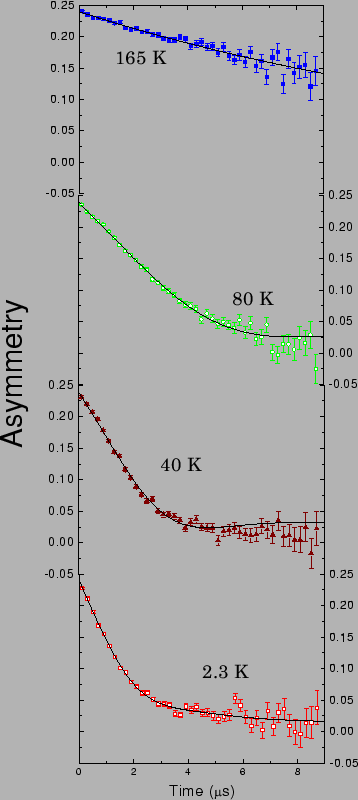.

Figure 4.1 shows the time evolution of the two-counter asymmetry at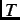=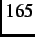K,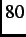K,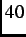K and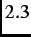K in the absence of an external magnetic field. The lack of any discrete frequency oscillations in the ZF-SR signals indicates that there is no onset of magnetic order at temperatures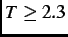K.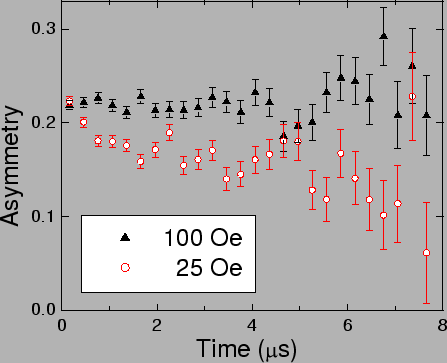To gain further insight into the nature of the magnetism which relaxes the ZF-SR signal, a constant magnetic field was applied along the direction of the initial muon-spin polarization. This arrangement is known as a longitudinal-field (LF)SR experiment. Muons implanted in the sample precesses about the local magnetic field, which is a vector sum of the applied field and the local internal magnetic fields due to nuclear and electronic magnetic moments. If the internal magnetic field is static and a large external magnetic field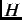is applied along the direction of the initial muon-spin polarization, the component of the resultant magnetic field perpendicular towill be small. Consequently the applied magnetic field decouples the muon spin from the local static magnetic fields . Figure 4.2 shows the time evolution of the asymmetry for applied longitudinal fields of=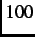Oe and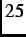Oe at=K. A longitudinal field ofOe is large enough to completely decouple the muon spin from the local magnetic field, evident by the non-relaxing asymmetry over the 8s time range. This indicates that the magnetism in the sample atK is fluctuating at a rate of less than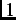MHz. As the Pr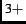ions have a non-magnetic singlet ground state and the local magnetic field due to the nuclear magnetic moments is small (i.e.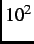-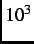times smaller than that of the electronic magnetic moments), the dominant source of local magnetism must be from Cu spins.

The solid curves in Fig. 4.1 are fits to the following relaxation function .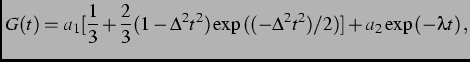(5.1)

where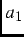+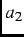=, and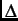and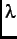are the Gaussian and exponential relaxation rates, respectively. Assuming that there is only one muon stopping site, the first term corresponds to the relaxation of the muon-spin polarization due to muons seeing only nuclear dipole moments. The second term is the relaxation due to muons which see dilute magnetic moments or magnetic clusters. Figure 4.3 shows the temperature dependence ofand. The exponential relaxation (i.e.= 0) at high temperature is likely due to fast'' fluctuating Cu spins, although this has not been verified by LF-SR measurements. At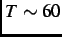K,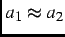. This indicates that the dilute magnetic moments are found in 50% of the sample volume. As the temperature is lowered,decreases andincreases, indicating that the dilute magnetic regions grow in size. Figure 4.4 shows the temperature dependence of the relaxation ratesand. As the temperature is lowered, the relaxation rates increase monotonically. This is consistent with a broadening of the magnetic field distribution due to gradual slowing down of the Cu spins.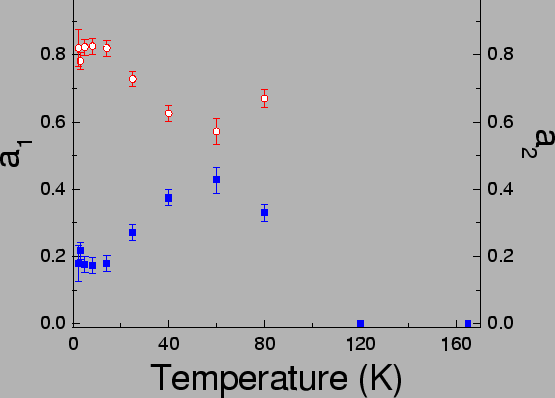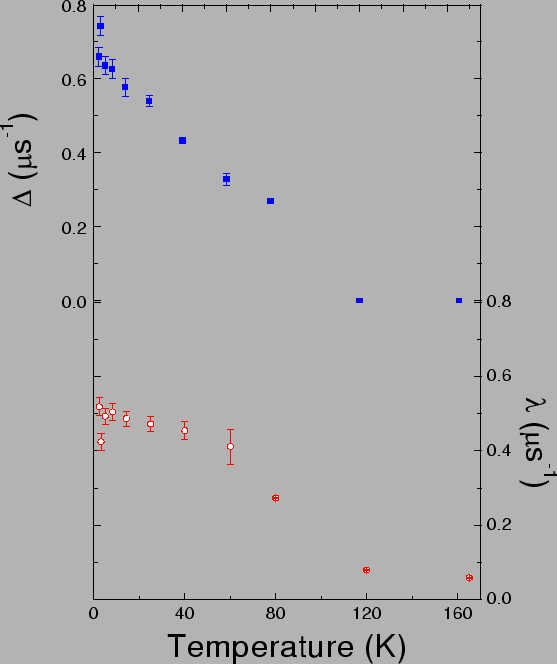Next: Transverse-Field Measurements Up: SR Studies of the Previous: Experimental Setup   Contents
Jess H. Brewer 2003-07-01